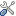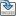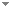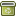The Jordan curve theorem and the Schönflies theorem in weak second-order arithmetic

Archive for Mathematical Logic 46 (5-6):465-480 (2007)

 Abstract In this paper, we show within ${\mathsf{RCA}_0}$ that both the Jordan curve theorem and the Schönflies theorem are equivalent to weak König’s lemma. Within ${\mathsf {WKL}_0}$ , we prove the Jordan curve theorem using an argument of non-standard analysis based on the fact that every countable non-standard model of ${\mathsf {WKL}_0}$ has a proper initial part that is isomorphic to itself (Tanaka in Math Logic Q 43:396–400, 1997) Keywords Second order arithmetic  Reverse mathematics  The Jordan curve theorem  The Schönflies theorem  Non-standard analysis Categories (categorize this paper) DOI 10.1007/s00153-007-0050-6 OptionsEdit this recordMark as duplicateExport citationFind it on ScholarRequest removal from indexRevision history

PhilArchive copy

Upload a copy of this paper     Check publisher's policy     Papers currently archived: 65,784

Setup an account with your affiliations in order to access resources via your University's proxy server
Configure custom proxy (use this if your affiliation does not provide a proxy)

References found in this work BETA

Subsystems of Second-Order Arithmetic.Stephen G. Simpson - 2004 - Studia Logica 77 (1):129-129.
The Self-Embedding Theorem of WKL0 and a Non-Standard Method.Kazuyuki Tanaka - 1997 - Annals of Pure and Applied Logic 84 (1):41-49.
Fixed Point Theory in Weak Second-Order Arithmetic.Naoki Shioji & Kazuyuki Tanaka - 1990 - Annals of Pure and Applied Logic 47 (2):167-188.

Citations of this work BETA

Erna and Friedman's Reverse Mathematics.Sam Sanders - 2011 - Journal of Symbolic Logic 76 (2):637 - 664.
Formalizing Non-Standard Arguments in Second-Order Arithmetic.Keita Yokoyama - 2010 - Journal of Symbolic Logic 75 (4):1199-1210.
Complex Analysis in Subsystems of Second Order Arithmetic.Keita Yokoyama - 2007 - Archive for Mathematical Logic 46 (1):15-35.
Tanaka’s theorem revisited.Saeideh Bahrami - 2020 - Archive for Mathematical Logic 59 (7-8):865-877.

Similar books and articles

Non-Standard Analysis in ACA0 and Riemann Mapping Theorem.Keita Yokoyama - 2007 - Mathematical Logic Quarterly 53 (2):132-146.
Complex Analysis in Subsystems of Second Order Arithmetic.Keita Yokoyama - 2007 - Archive for Mathematical Logic 46 (1):15-35.
A Nonstandard Counterpart of WWKL.Stephen G. Simpson & Keita Yokoyama - 2011 - Notre Dame Journal of Formal Logic 52 (3):229-243.
How Incomputable Is the Separable Hahn-Banach Theorem?Guido Gherardi & Alberto Marcone - 2009 - Notre Dame Journal of Formal Logic 50 (4):393-425.
Classifying Dini's Theorem.Josef Berger & Peter Schuster - 2006 - Notre Dame Journal of Formal Logic 47 (2):253-262.
The Dirac Delta Function in Two Settings of Reverse Mathematics.Sam Sanders & Keita Yokoyama - 2012 - Archive for Mathematical Logic 51 (1-2):99-121.
Some More Conservation Results on the Baire Category Theorem.Takeshi Yamazaki - 2000 - Mathematical Logic Quarterly 46 (1):105-110.
Reverse Mathematics and a Ramsey-Type König's Lemma.Stephen Flood - 2012 - Journal of Symbolic Logic 77 (4):1272-1280.